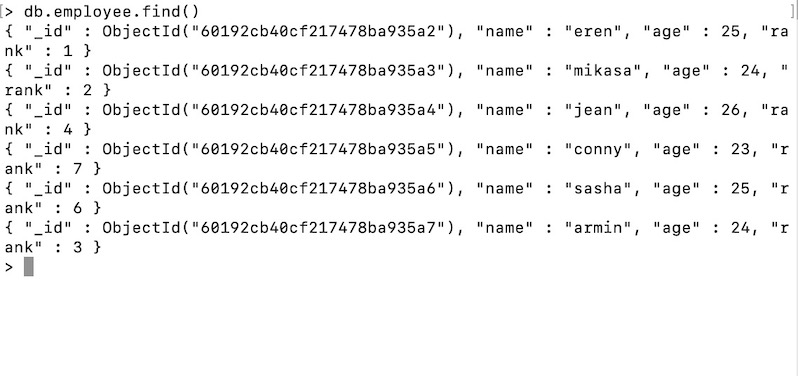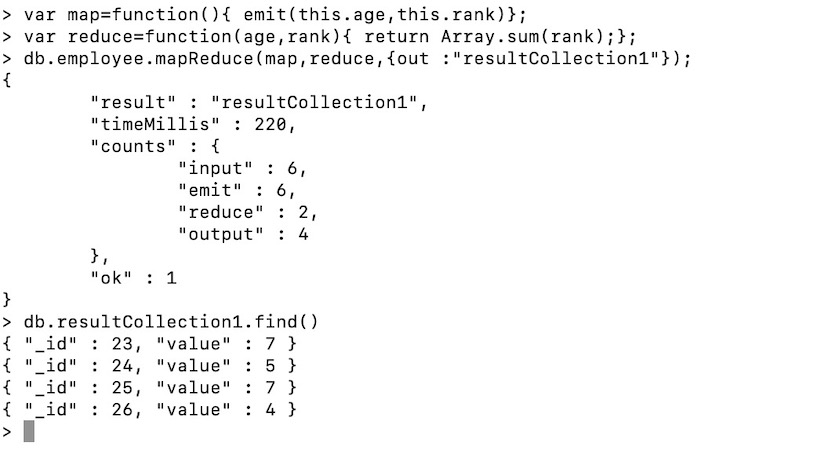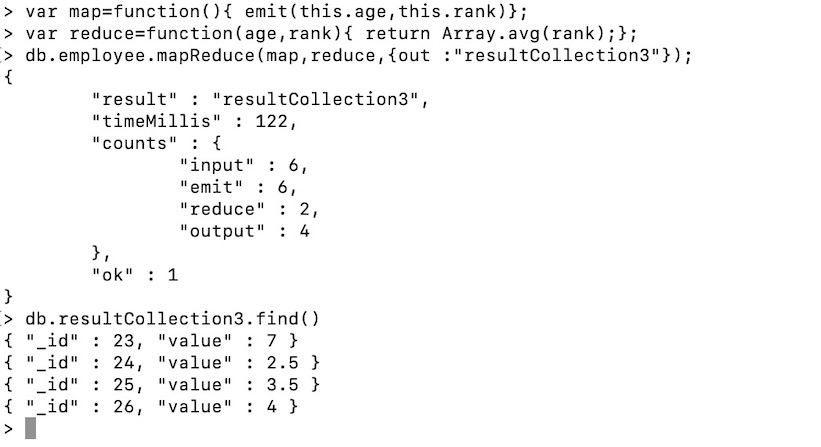Related Articles
MongoDB – Map Reduce
• Last Updated : 05 Feb, 2021

In MongoDB, map-reduce is a data processing programming model that helps to perform operations on large data sets and produce aggregated results. MongoDB provides the mapReduce() function to perform the map-reduce operations. This function has two main functions, i.e., map function and reduce function. The map function is used to group all the data based on the key-value and the reduce function is used to perform operations on the mapped data. So, the data is independently mapped and reduced in different spaces and then combined together in the function and the result will save to the specified new collection. This mapReduce() function generally operated on large data sets only. Using Map Reduce you can perform aggregation operations such as max, avg on the data using some key and it is similar to groupBy in SQL. It performs on data independently and parallel. Let’s try to understand the mapReduce() using the following example:

In this example, we have five records from which we need to take out the maximum marks of each section and the keys are id, sec, marks.

```{"id":1, "sec":A, "marks":80}
{"id":2, "sec":A, "marks":90}
{"id":1, "sec":B, "marks":99}
{"id":1, "sec":B, "marks":95}
{"id":1, "sec":C, "marks":90}```

Here we need to find the maximum marks in each section. So, our key by which we will group documents is the sec key and the value will be marks. Inside the map function, we use emit(this.sec, this.marks) function, and we will return the sec and marks of each record(document) from the emit function. This is similar to group By MySQL.

`var map = function(){emit(this.sec, this.marks)};`

After iterating over each document Emit function will give back the data like this:

{“A”:[80, 90]},  {“B”:[99, 90]},  {“C”: }

and upto this point it is what map() function does. The data given by emit function is grouped by sec key, Now this data will be input to our reduce function. Reduce function is where actual aggregation of data takes place. In our example we will pick the Max of each section like for sec A:[80, 90] = 90 (Max)  B:[99, 90] = 99 (max) , C: = 90(max).

`var reduce = function(sec,marks){return Array.max(marks);};`

Here in reduce() function, we have reduced the records now we will output them into a new collection.{out :”collectionName”}

`db.collectionName.mapReduce(map,reduce,{out :"collectionName"});`

In the above query we have already defined the map, reduce. Then for checking we need to look into the newly created collection we can use the query db.collectionName.find() we get:

```{"id":"A", value:90}
{"id":"B", value:99}
{"id":"C", value:90}```

Syntax:

```db.collectionName.mapReduce(
... map(),
...reduce(),
...query{},
...output{}
);```

Here,

• map() function: It uses emit() function in which it takes two parameters key and value key. Here the key is on which we make groups like groups by in MySQL. Example like group by ages or names and the second parameter is on which aggregation is performed like avg(), sum() is calculated on.
• reduce() function: It is the step in which we perform our aggregate function  like avg(), sum().
• query: Here we will pass the query to filter the resultset.
• output: In this, we will specify the collection name where the result will be stored.

Example 1:

In this example, we are working with:

Database: geeksforgeeks2

Collection: employee

Documents: Six documents that contains the details of the employees• Find the sum of ranks grouped by ages:
```var map=function(){ emit(this.age,this.rank)};
var reduce=function(age,rank){ return Array.sum(rank);};
db.employee.mapReduce(map,reduce,{out :"resultCollection1"});```

Here, we will calculate the sum of rank present inside the particular age group. Now age is our key on which we will perform group by (like in MySQL) and rank will be the key on which we will perform sum aggregation.

• Inside map() function, i.e., map() : function map(){ emit(this.age,this.rank);}; we will write the emit(this.age,this.rank) function. Here this represents the current collection being iterated and the first key is age using age we will group the result like having age 24 give the sum of all rank or having age 25 give the sum of all rank and the second argument is rank on which aggregation will be performed.
• Inside the reduce function, i.e., reduce(): function reduce(key,rank){ return Array.sum(rank); }; we will perform the aggregation function.
• Now the third parameter will be output where we will define the collection where the result will be saved, i.e., {out :”resultCollection1″}. Here, out represents the key whose value is the collection name where the result will be saved.• Performing avg() aggregation on rank grouped by ages:
```var map=function(){ emit(this.age,this.rank)};
var reduce=function(age,rank){ return Array.avg(rank);};
db.employee.mapReduce(map,reduce,{out :"resultCollection3"});
db.resultCollection3.find()```

In this example, we will calculate the average of the ranks grouped by age. So,

• map(): Function map(){ emit(this.age, this.rank)};. Here age is the key by which we will group and rank is the key on which avg() aggregation will be performed.
• reduce(): Function reduce (age,rank){ return Array.avg(rank)l};
• output: {out:”resultCollection3″}## When to use Map-Reduce?

In MongoDB, you can use Map-reduce when your aggregation query is slow because data is present in a large amount and the aggregation query is taking more time to process. So using map-reduce you can perform action faster than aggregation query.

My Personal Notes arrow_drop_up